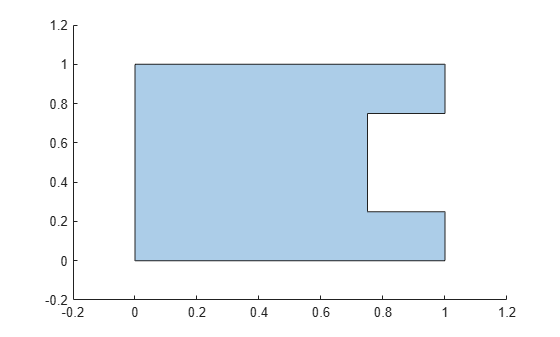Documentation

### This is machine translation

Mouseover text to see original. Click the button below to return to the English version of the page.

To view all translated materials including this page, select Country from the country navigator on the bottom of this page.

# subtract

Difference of two `polyshape` objects

## Syntax

``polyout = subtract(poly1,poly2)``
``[polyout,shapeID,vertexID] = subtract(poly1,poly2)``
``___ = subtract(poly1,poly2,'KeepCollinearPoints',TF)``

## Description

example

````polyout = subtract(poly1,poly2)` returns a `polyshape` object whose regions are the geometric difference of two `polyshape` objects. The output `polyout` contains the regions of `poly1` minus any part of `poly2` that overlaps with `poly1`. The input arguments `poly1` and `poly2` must have compatible array sizes.```

example

````[polyout,shapeID,vertexID] = subtract(poly1,poly2)` also returns vertex mapping information from the vertices in `polyout` to the vertices in `poly1` and `poly2`. The `subtract` function only supports this syntax when `poly1` and `poly2` are scalar `polyshape` objects.The `shapeID` elements identify whether the corresponding vertex in `polyout` originated in `poly1`, `poly2`, or was created from the difference. `vertexID` maps the vertices of `polyout` to the vertices of `poly1`, `poly2`, or the difference.```
````___ = subtract(poly1,poly2,'KeepCollinearPoints',TF)` specifies whether to keep or remove collinear points in `polyout` for any of the previous syntaxes.```

## Examples

collapse all

Create and plot two polygons `poly1` and `poly2` that partially overlap.

```poly1 = polyshape([0 0 1 1],[1 0 0 1]); poly2 = polyshape([0.75 1.25 1.25 0.75],[0.25 0.25 0.75 0.75]); plot(poly1) hold on plot(poly2)````figure`

Subtract `poly2` from `poly1`. The resulting polygon is `poly1` minus any part of `poly2` that overlaps with `poly1`.

`polyout1 = subtract(poly1,poly2)`
```polyout1 = polyshape with properties: Vertices: [8x2 double] NumRegions: 1 NumHoles: 0 ```
`plot(polyout1)`Now subtract the two polygons in the opposite order, that is, subtract `poly1` from `poly2`. The resulting polygon is `poly2` minus any part of `poly1` that overlaps `poly2`.

`polyout2 = subtract(poly2,poly1)`
```polyout2 = polyshape with properties: Vertices: [4x2 double] NumRegions: 1 NumHoles: 0 ```
```plot(polyout2) xlim([-0.2 1.4]); ylim([-0.2 1.2]);```Create two polygons, and compute and plot their difference. Display the vertex coordinates of the difference and the corresponding vertex mapping information.

```poly1 = polyshape([0 0 1 1],[1 0 0 1]); poly2 = translate(poly1,[0.5 0.5]); [polyout,shapeID,vertexID] = subtract(poly1,poly2); plot(polyout) axis equal````[polyout.Vertices shapeID vertexID]`
```ans = 6×4 0 1.0000 1.0000 1.0000 0.5000 1.0000 0 0 0.5000 0.5000 2.0000 4.0000 1.0000 0.5000 0 0 1.0000 0 1.0000 3.0000 0 0 1.0000 4.0000 ```

The first, fifth, and sixth vertices of the difference originated in `poly1`, since the corresponding values in `shapeID` are 1. These vertices are the first, third, and fourth vertices in the property `poly1.Vertices`, respectively, since the corresponding values in `vertexID` are 1, 3, and 4. Similarly, the third vertex of the difference originated in `poly2`, and is the fourth vertex in the property `poly2.Vertices`. The second and fourth vertices of the difference were created from the subtraction computation because the corresponding values of `shapeID` and `vertexID` are 0.

## Input Arguments

collapse all

First input `polyshape`, specified as a scalar, vector, matrix, or multidimensional array.

Second input `polyshape`, specified as a scalar, vector, matrix, or multidimensional array.

Collinear vertices indicator, specified as `false` or `true`:

• `false` — Remove collinear points so that the output `polyshape` contains the fewest vertices necessary to define the boundaries.

• `true` — Keep all collinear points as vertices.

When the `'KeepCollinearPoints'` parameter is not specified, its value is assigned according to the values used when creating the input `polyshape` objects:

• If the value was `true` for each input `polyshape` when they were created, then the value for the output `polyshape` is set to `true`.

• If the value was `false` for each input `polyshape` when they were created, then the value for the output `polyshape` is set to `false`.

• If the values for the input `polyshape` objects do not match, then the value for the output `polyshape` is set to `false`.

Data Types: `logical`

## Output Arguments

collapse all

Output `polyshape`, returned as a scalar, vector, matrix, or multidimensional array.

The two input `polyshape` arguments must have compatible sizes. For example, if two input `polyshape` vectors have different lengths M and N, then they must have different orientations (one must be a row vector and one must be a column vector). `polyout` is then M-by-N or N-by-M depending on the orientation of each input vector. For more information on compatible array sizes, see Compatible Array Sizes for Basic Operations.

Shape ID, returned as a column vector whose elements each represent the origin of the vertex in the difference. The value of an element in `shapeID` is 0 when the corresponding vertex of the output `polyshape` was created by the subtraction. An element is 1 when the corresponding vertex originated from `poly1`, and 2 when it originated from `poly2`.

The length of `shapeID` is equal to the number of rows in the `Vertices` property of the output `polyshape`. The `xor` function only supports this output argument if the input `polyshape` objects are scalar.

Data Types: `double`

Vertex ID, returned as a column vector whose elements map the vertices in the output `polyshape` to the vertices in the `polyshape` of origin. The elements of `vertexID` contain the row numbers of the corresponding vertices in the `Vertices` property of the input `polyshape`. An element is 0 when the corresponding vertex of the output `polyshape` was created by the difference.

The length of `vertexID` is equal to the number of rows in the `Vertices` property of the output `polyshape`. The `subtract` function only supports this output argument when the input `polyshape` objects are scalar.

Data Types: `double`